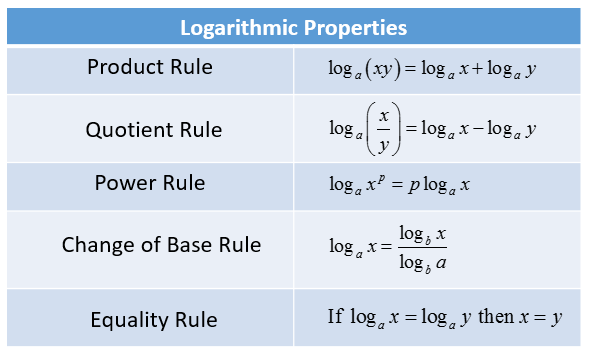# Unit 5!

` In this unit we will be discussing Expanding logarithmic functions, condensing logarithmic functions, solving exponential and logarithmic equations`

When expanding logarithms we must take into account of the different rules that come with it.log6(5x^3/y)

### Step 2: Quotient, Product, Equality, & Change of base rules.

Note: When there is division in the logarithm then you must subtract the log of the divisor from the log of the dividend, when using multiplication you add both logs.

log6(5) + log6(x^3) - log6(y)

As you can see here we're literally just expanding the equation by attaching log6() to every piece

### Step 3: Power Rule

When using this property, you move any exponent to the front of the variable by which the exponent is attached to

log6(x^3) = 3log6(x)

### Step 4: Write out final expanded equation

log6(5) + 3log6(x) - log6(y)